Kyu Jun Cho (kc499)

Sung-kyoon Im (si63)

Jaehoon Kim (jk828)

ECE4760

Final Project : “Invisible Band”

Introduction  <to the top>

The goal of this project is designing microcontroller operated drum set and guitar which are only consist of drums sticks, pedals, and pick. This is done by implementing accelerometers which are connected to the microcontroller. By swinging the sticks and picking the guitar in the air you can play drums and guitar. We also implemented playback feature that you can record the sound and listen to it later. Rock n Roll!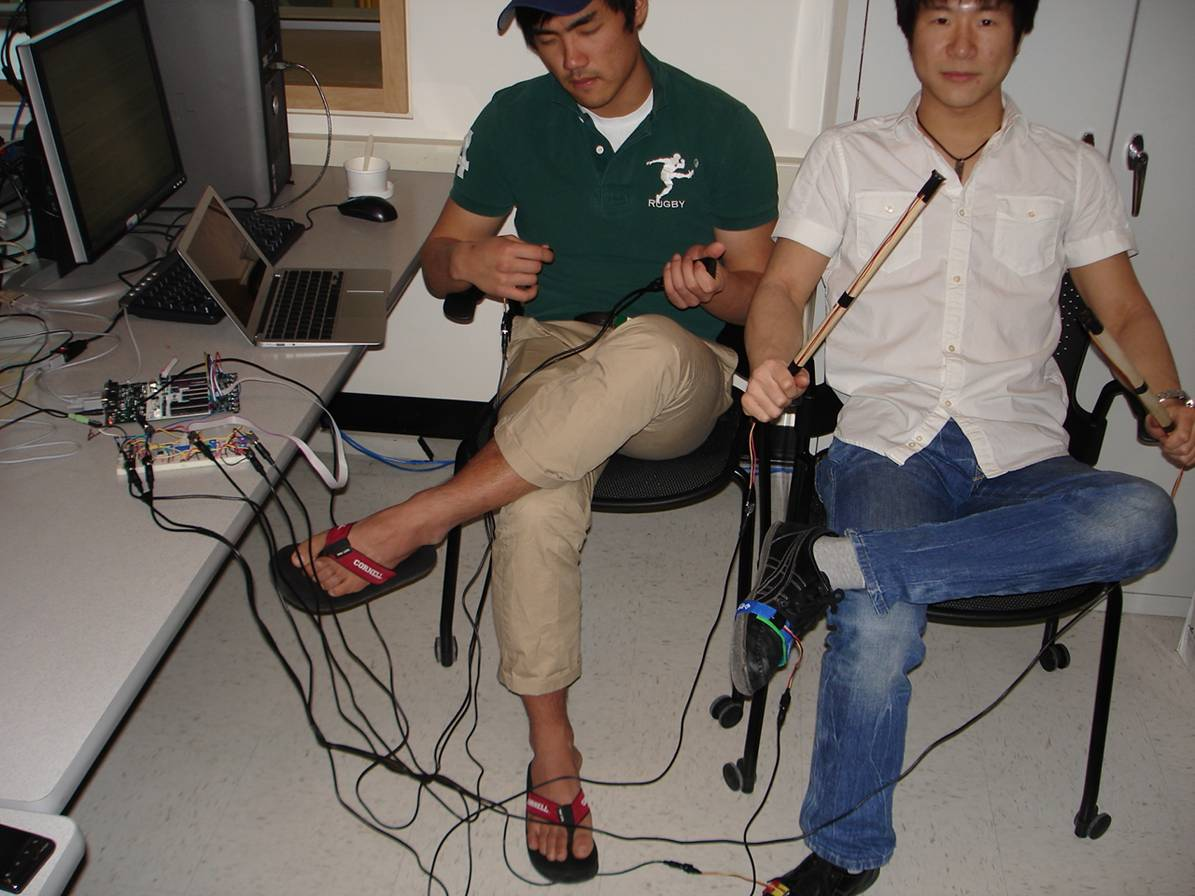High level design  <to the top>

-Block diagram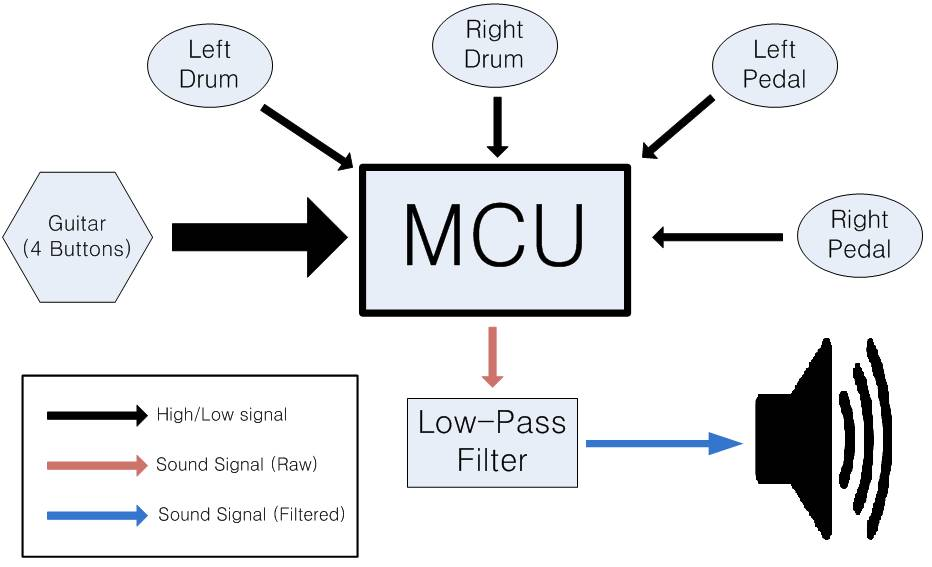Figure 1 – Block diagram

Swinging drum sticks is essentially applying acceleration to it. This is detected by accelerometer which generates higher voltage relative to the steady state. When this “High” voltage output is detected by MCU it will generate sound using Karplus-Strong algorithm (for guitar) and FM synthesis (for drum), the theory of which will be explained in the following section. This “Raw” sound output then goes through the low-pass filter to suppress some unnecessary high frequency components and we can listen to the filtered sound through the speaker.

-Sound Implementation

A guitar consists of several strings that fluctuate when they are plucked. The fluctuation is what makes the sound, and this is why the Karplus-Strong Algorithm is one of the best ways to implement the guitar sound. Karplus-Strong Algorithm fills a delay line, a circular buffer, with noise, and then lowpass filters filter the shifted outputs of the delay line and adds those to themselves. The noise table will change the pluck transient timber.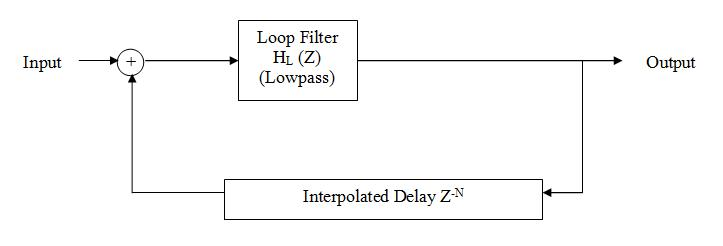Figure2 – Karplus-Strong Algorithm

Since the Karpls-Strong Algorithm is essentially a physical modeling of string simulation sounds other than string instruments is not so well. Therefore, to simulate the sounds of other instrument, the FM modulation of a sine wave should be introduced. The basic structure is same. The main function will set up the flags for each sound whenever it gets signal, and the sounds are played in ISR. The difference is that in FM Synthesis, only one array of sine wave will be needed, since the sounds will be differed by the base and FM frequency.

Hardware Design  <to the top>

-Drum sticks and pedals

For this project we used MMA1260D accelerometer (shown below) which has detection range of ±1.5g. (Acceleration of gravity, g= 9.8m/s2)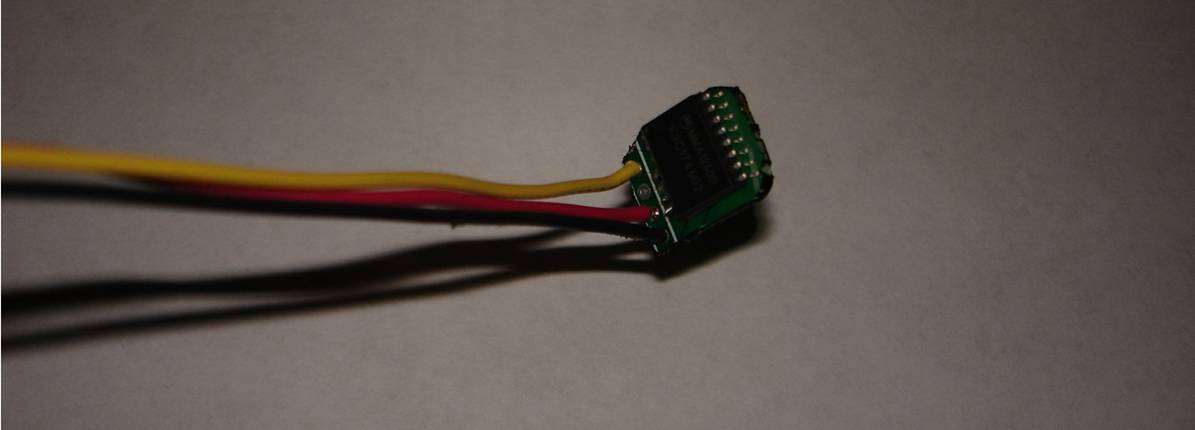Figure3 – MMA1260D Accelerometer (Vcc: red wire, GND: black wire, Output: yellow wire)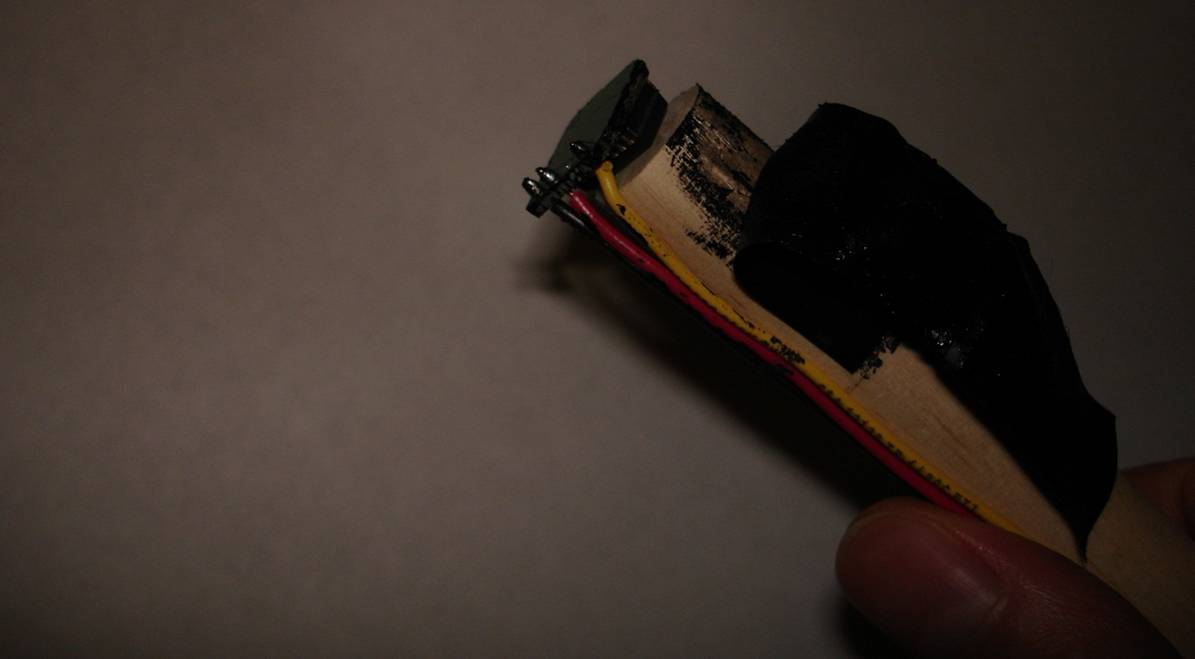Figure 4 – Tip of drum stick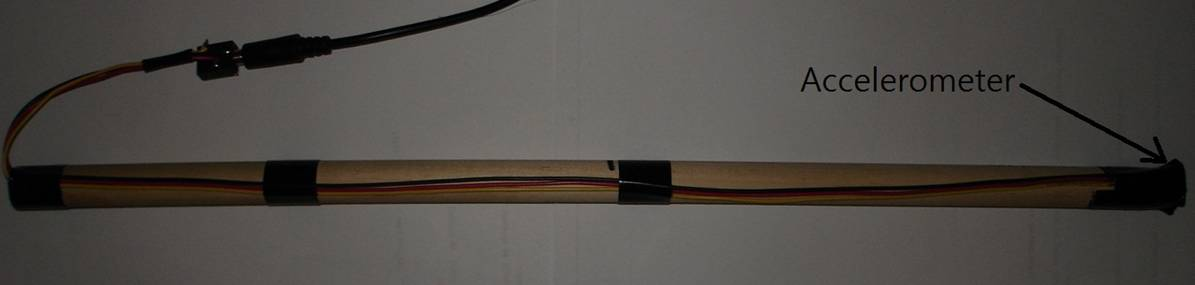Figure 5 – Drum stick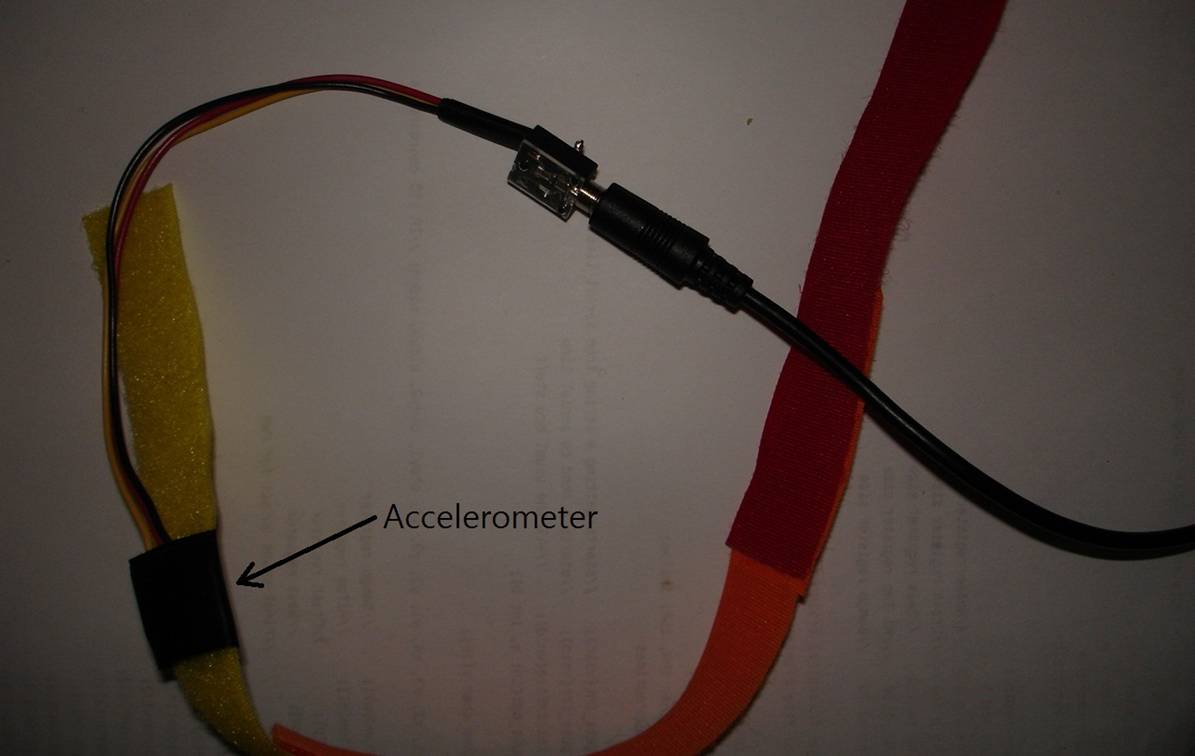Figure 6 –Pedal (R)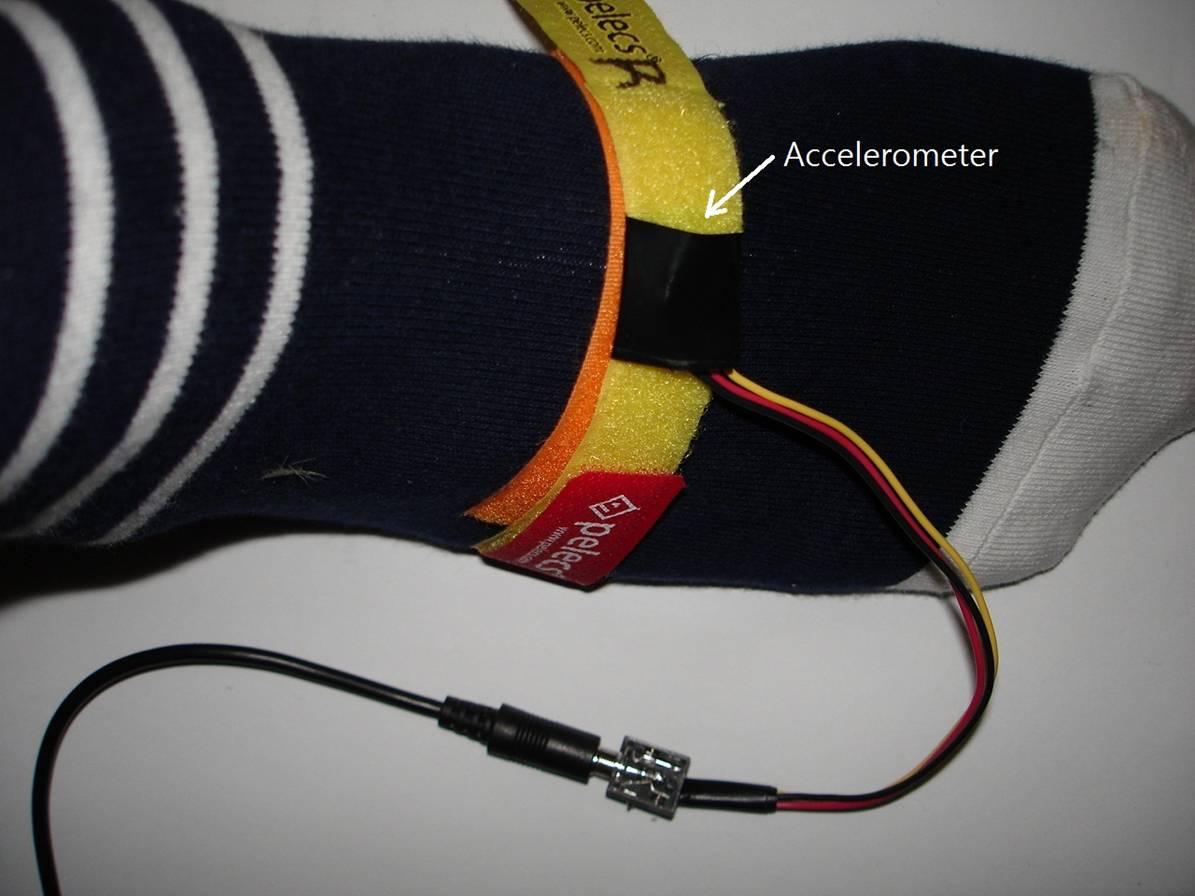Figure 7 –Pedal strapped around Sung-kyoon’s foot (R)

Accelerometers are mounted on drumsticks and pedals and accelerometer output goes to LM358 comparator which generates high (typically around 3.5v) or low (typically around 0.5v) voltage depending on threshold voltage. Threshold voltage is directly related to the sensitivity of drumstick because, for example, higher threshold voltage requires higher acceleration voltage output in order for LM358 comparator to be in high voltage.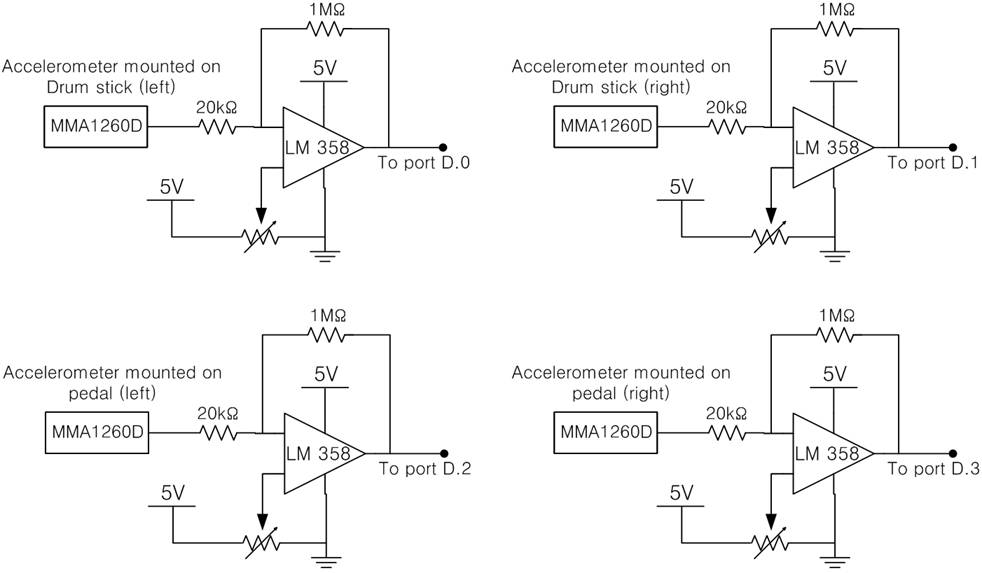Figure 8 – Circuit diagram for sticks and pedals

Threshold voltage can be adjusted by changing variable resistances (trimpod) shown on the circuit diagrams above. Turning trimpod counter clockwise will reduce the threshold voltage and increase it otherwise. We used about 3v for drumstick and 2v for pedal.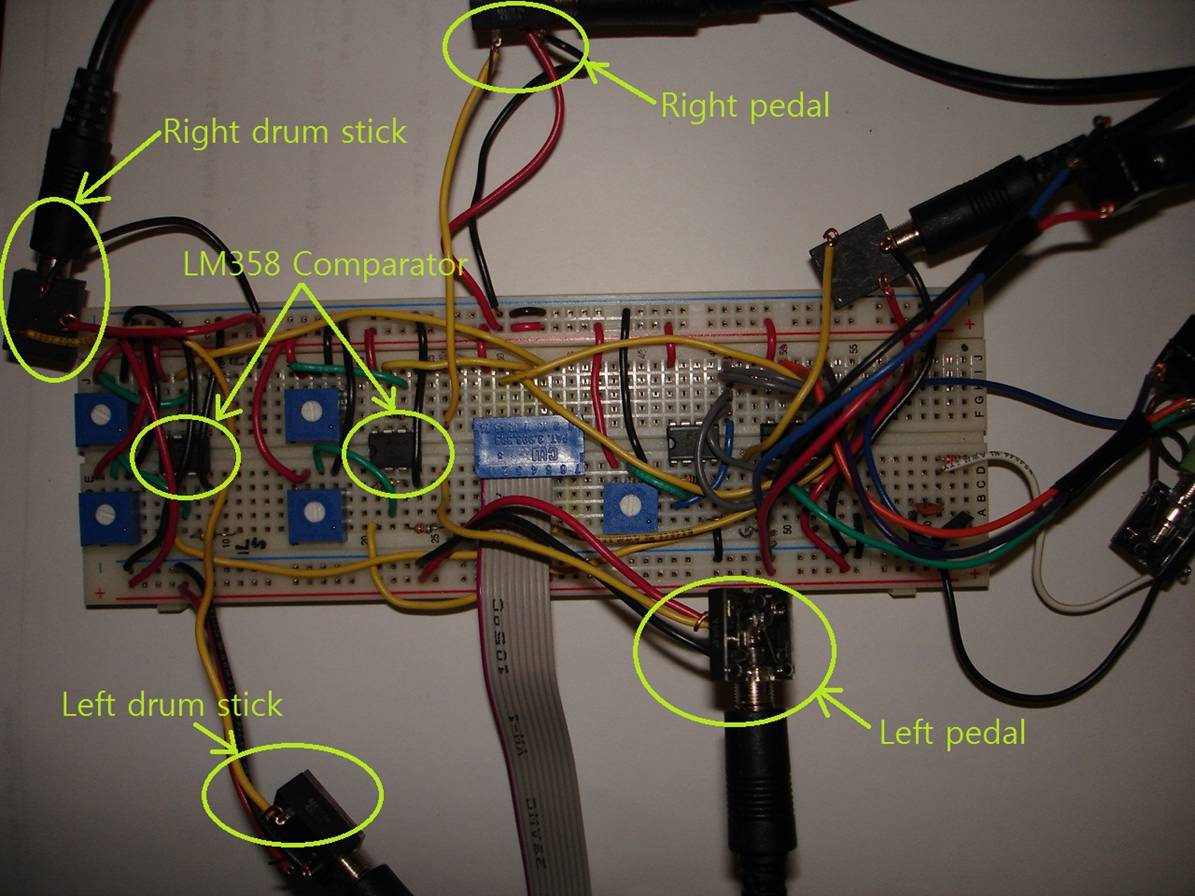Figure 9 – Protoboard (sticks and pedal inputs are shown)

-Guitar

Guitar is consists of two parts: pick and “neck” which determines the sound.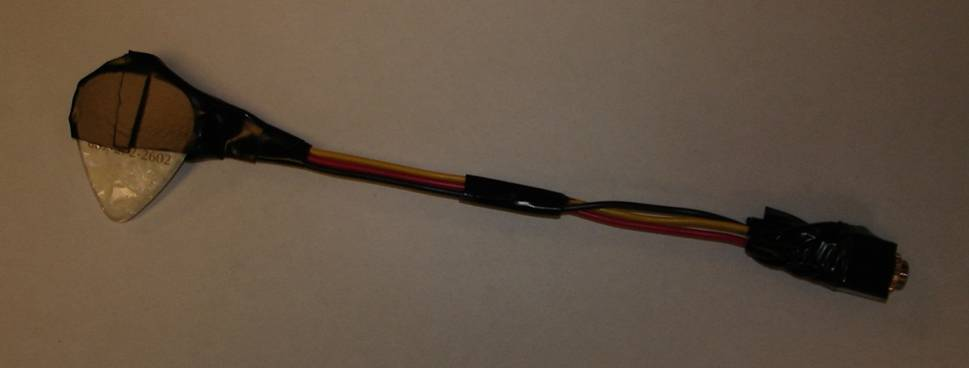Figure 10 – Pick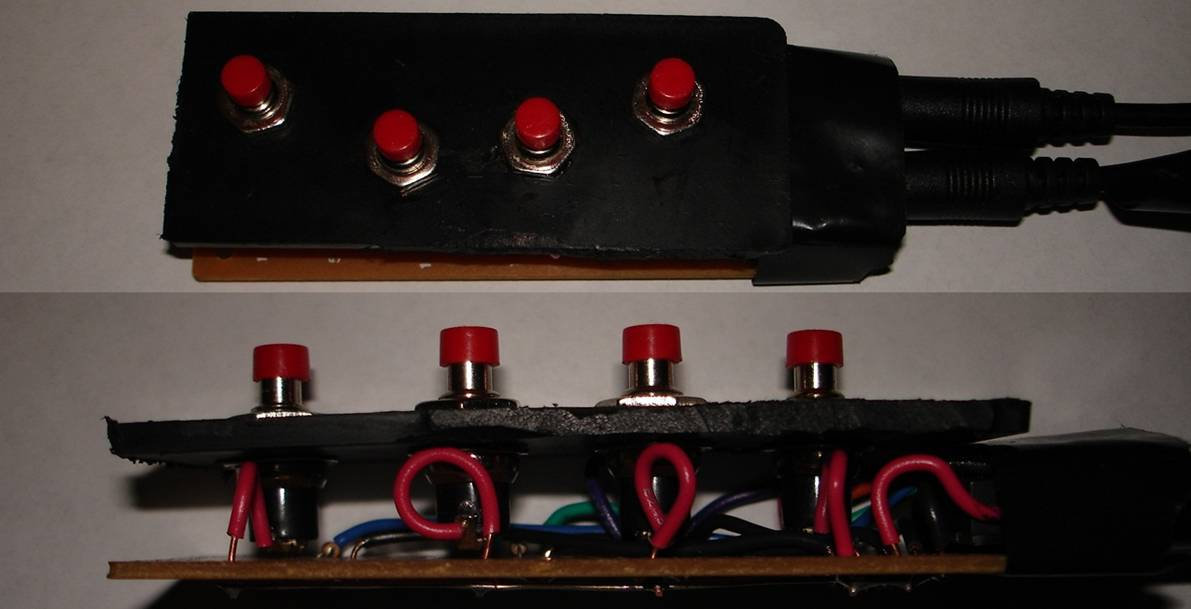Figure 11 – “Neck”

Pick is fundamentally the same as the drum stick already explained because it is an accelerometer attached to the actual guitar pick (decoration purpose). “Neck” has four buttons which corresponds to different guitar sounds. Pressing these buttons, however, won’t produce any sound. You have to stroke the pick while pressing the button(s). The following circuit diagram explains how “neck” and pick are connected.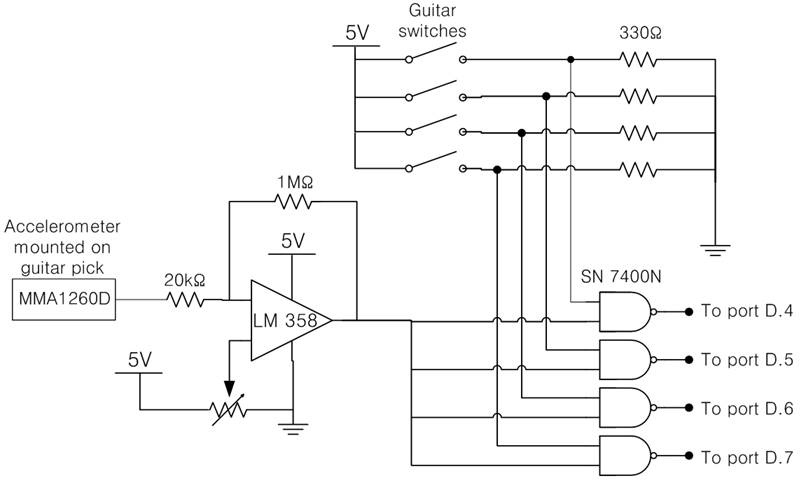Figure 12 – Circuit diagram for guitar

The left half of the circuit diagram is the same as that of drum stick; when you stroke the pick hard enough for accelerometer to produce voltage higher than threshold voltage set by variable resistor connected to LM358 comparator it will produce logic high voltage which goes to each of NAND gate shown above. Red buttons in figure 11 corresponds to Guitar switches shown above circuit diagram. When button is pressed the switch is closed and logic high voltage goes to corresponding NAND gate. So if the user strokes the pick while button is pressed the output of NAND gate that corresponds to the button become logic low. Then MCU reads this signal and plays the corresponding sound. 330Ω resistor was used to pull down the voltage that goes into the NAND gate when the switch is open. Choosing right resistance is important because the input when the switch is open (logic low) has to be lower than 0.8v (refer to SN7400N spec sheet) or it will read as logic high. By having 330Ω resistor we were able to maintain voltage around 0.3v when switch is open.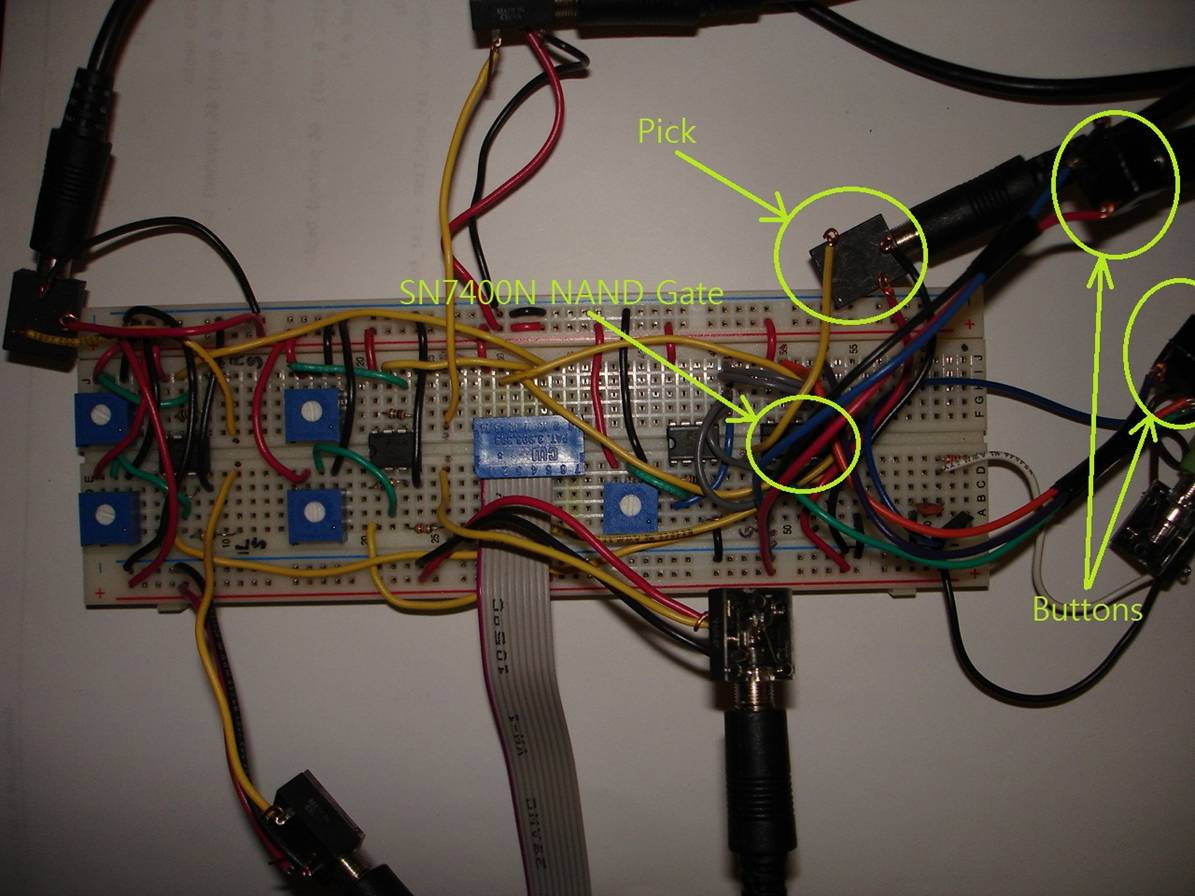Figure 13 – Protoboard (guitar inputs(pick and buttons) are shown)

-Low-Pass filter

To reduce undesired high frequency component of sound we implemented a low-pass filter, a simple combination of capacitor and resistor. Cutoff frequency, fc, is defined as fc= 1/(2πRC). We chose R=1kΩ and C=45nf to set fc to be around 3500Hz. Because ISR is running with 8000Hz that the highest frequency that will be generated is 4000Hz. And it is clear that 4000Hz is unlikely to be generated that cutoff frequency of 3500Hz is reasonable choice.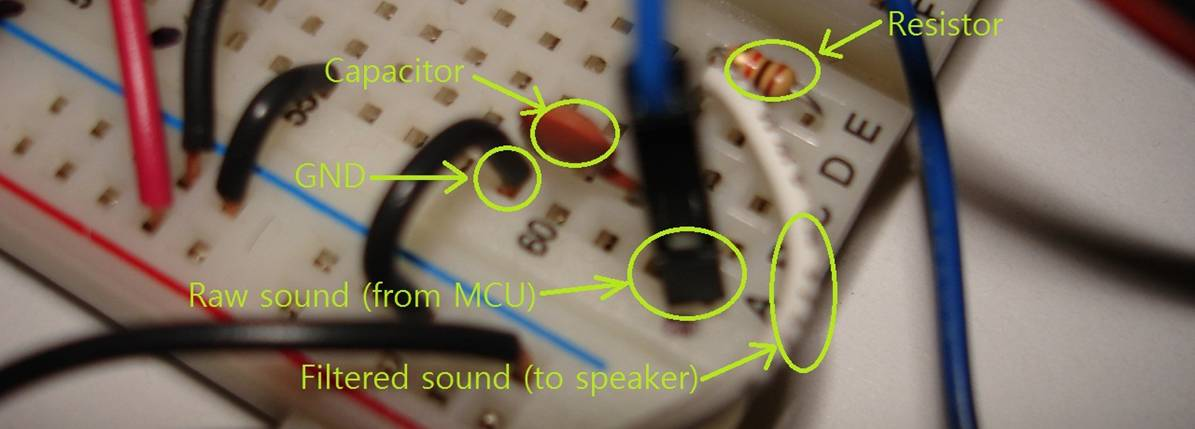Figure 14 – Low-pass filter

-Connecting with 3.5mm audio cables

We used seven 3.5mm audio cables and fourteen jacks to make assembling and disassembling the circuit easy. 3.5mm cable was specifically chosen because it is consists of three wires which can be used as Vcc, GND, and output voltage when connecting accelerometer to the protoboard. Also 3.5mm audio cable is more flexible than using three conventional wires that playing drum is much better with 3.5 mm audio cable.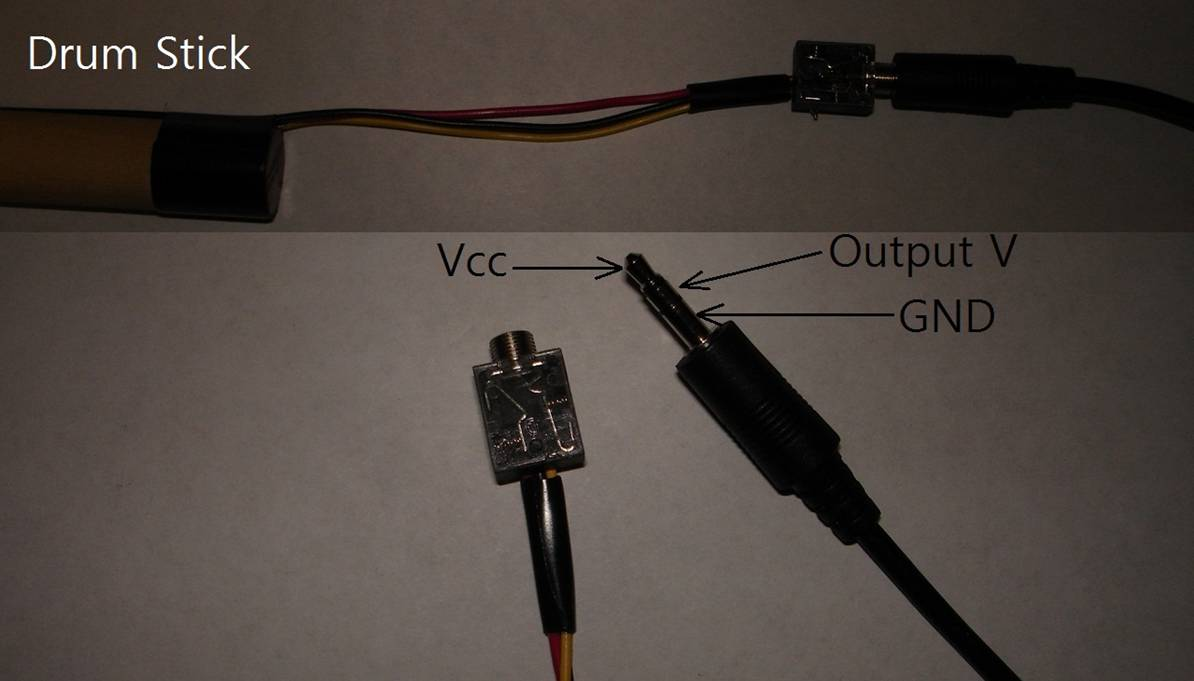Figure 15 – Usage of 3.5mm audio cables (accelerometer output)

Two 3.5mm audio cables were used to connect “neck” with the protoboard because “neck” requires six wires: Vcc, GND, and 4 button signals. Top picture of figure 3 shows two audio cables are connected to “neck”.

-Buttons and LEDs

To minimize the cost we used built-in switches and LEDs on STK500 as buttons and status indicators. Each of their functions is described in the table below.

 Switch function LED function SW0 Recording/Stop LED0 Turns on when recording. Turns off when recording stops SW1 Playback LED1 Turns on during playback. Turns off when playback is done. SW2 Changes damping time of guitar LED2 Turns on in short damping mode. Turns off in normal mode. SW3 Changes string combination of guitar LED3 Sound combination 1 : light Sound combination 2 : no light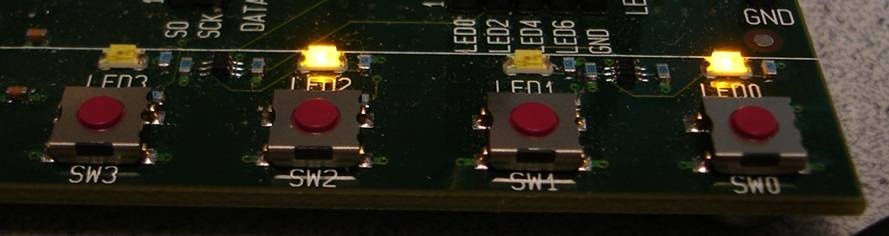Figure 16 – Built-in switches and LEDs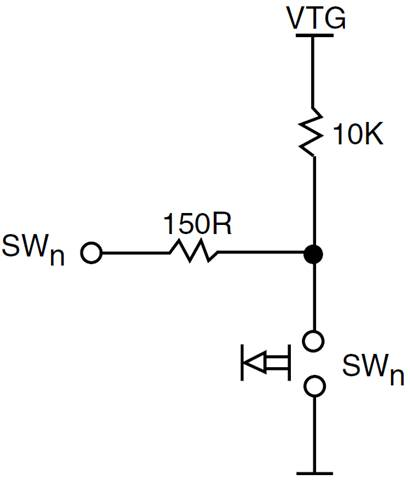Figure 17 – Built-in switch on STK500

We used SW0 (connected to C.0) as record/ stop button and SW1 (connected to C.1) as playback button. Pressing SW2 changes the damping time of guitar sound which

Software Design

-Basic Structure

We used Karplus-Strong Algorithm to implement the sound of guitar while we used FM Synthesis Algorithm to implement that of drum. Since both algorithms consist of similar structure, we could implement both sounds concurrently with two basic functions, main function and ISR. Two functions will include the codes for both guitar and drum.

The main function accepts the signals from the invisible drum, the invisible guitar, and switch for recording and replaying. The main function first initializes all the parameters for playing different sounds and flags for ISR. Then, the main function enters the infinite while loop. In the while loop, the first thing done is to check if there is any note is played the invisible instruments. It then checks if there is any button is pushed for recording and replaying. If there is any key played, it debounces the signal and set the flag up for the ISR to play the sound. It also sets up the LED so that if the sounds are being recorded or replayed. No LED is on if it is just being played.

The Interrupt Service Routine updates all the sound parameters and arrays when the flags are up. It also calculates all the outputs and sends it to Port.B 3.

-Karplus-Strong Algorithm and FM Synthesis

The buffers are saved as arrays, named string1, string2, string3, and string4, in the code, and the length of each array determines the sound. The timber is determined by the lowpass filter, which is saved as lowpass_out1, lowpass_out2, lowpass_out3, and lowpass_out4.

There are four buttons in the neck, so we need to implement four different guitar sounds for each button, four different set of pointers used for the string, ptrin1, ptrout1, ptrin2, ptrout2, ptrin3, ptrout3, ptrin4, ptrout4. Even though each button plays different sounds, they can share one noise table, so there will be only one noise table. The main function will accept the signals for the invisible guitar through Port.D 4, Port.D 5, Port.D 6 and Port.D 7. As soon as the main function get the signal, it debounces the signal and set the flag, pluck1, pluck2, pluck3, and pluck4. ISR runs 8000 times per second, so it has the frequency of 8000 Hz. ISR updates each string array whenever it gets the flag from the main function and turn off the flag. The flag does not have to be on for the whole time the sound is played. The string is updated when the flag is up, and the amplitude decays automatically. Therefore, if it is not flagged, each string will have the amplitude of 0, which will make no sound. In this way, we can add the sounds without worrying about which sound is played, and we can play sounds concurrently.

-Recording and Replaying

There are 8 arrays of length 100 for each sound, which will include at what time count each flag is up. For example, if the left drum sound is played at time = 200 when the recording is 1, the array for left drum will include 200. Saving the time count in each array is done when the flag for each sound is set to 1. The inputs from the switches are updated in the while loop in the main function. Pushing switch 0 will set the flag ‘recording’ to 1 when it was recording, and vice versa. When the replaying flag is on, the while loop will not update the inputs from the instruments. Instead, in ISR, as the counter increments, ISR checks if there is any array that matches the counter. If the counter matches the saved time, ISR sets the flag up for the sound. The index pointers for recording arrays and time counter are initialized whenever the recoding and replaying flags are set. Another important point is that ISR increments the time counter every 1/8000 second, and the maximum value unsigned integer can have is 2^16. Therefore, the time counter will reach its maximum value after (2^16)/8000 = 8.2 seconds. Therefore, we implemented the time counts so that the time is incremented every 0.1 second. Then the time counter will hit its maximum value at (2^16) / 10 = 6553 seconds, which is about 2 hours. In other words, our system can either save 100 flag for each sound or at most about 2 hours.

-Effect

The third button will flag the button for damping. If the third button is pressed, the damping parameters for the guitar sounds will be increased, which results in short sound. When the button is pressed, the flag becomes one just like the one for the recording. The third led will be also on so that the user can know that the effect is on.

-More notes

Since there are only four buttons, there are only four sounds that can be implemented. Therefore, we implemented another button that four different notes can be played when pushed. The four guitar buttons can originally play C, D, E and F. When the button is pushed and the flag is up, the button for C will play G, D will play A, E will play B, and F will play C (higher octave). The fourth button is for the higher pitch, and the fourth led is on when the buttons are playing higher notes.

* The more details about the parameters for sound, how we implemented flags, and how we handle the input signals from instruments and switch and output signals to led and speaker are in the code.

Result

Each sound can be played concurrently. Each sound can have different tunes and pitch depends on its parameters. Because there are not many instruments (and the microcontroller cannot handle many), it does not have enough notes to play the whole song, but it is still very playable. Due to the limits of the memory the microcontroller can handle, each recording array has the length of 100. In other words, each sound can be played 100 times. Therefore, at most 800 sounds can be recorded. The most significant aspect about our system is that you can change the replay sound by pushing buttons when it is being replayed. In this way, we can listen to what we record with different damping, or note. It is possible that recorded array can be saved in other locations.

Conclusion

Our original proposal for this project is slightly different from our current design. Originally we planned to only make a drum set. We were able to successfully make a drum set, and in addition, we small portion of guitar and also added features such as recording and replaying, sound effect, and changing note for give button.

This project is something everyone can play and enjoy. This project has the potential to “evolve” into many different ways, such as video game and full guitar set.

One further improvement we believe we can make is to use more switch button to add sound effect. Adding effect such as distortion and delay would make this project more “real- like” and ultimately more fun.

Appendix

-Cost

 Items Part Number Source Quantity Unit Price Total Price Wooden stick (drum) Cornell Store 1 0.62 0.62 Straps (pedal) Pre-owned 6 trimpod Lab 5 Accelerometer MMA1260D Lab 5 Comparator LM358 Lab 3 Resistor (20kΩ) Lab 5 Resistor (1MΩ) Lab 5 Resistor (330Ω) Lab 4 Resistor (1kΩ) Lab 1 Capacitor (45F) Lab 1 NAND gates SN7400N Lab 1 3.5mm audio cable Lab 7 1.25 8.75 3.5mm audio jack Lab 15 0.50 7.50 Protoboard Lab 1 Push Button Lab 4 4 4 Solder Board Lab 1 4 4 Speaker Lab 1 STK500 Lab 1 15 15 Jumper cable Lab 1 9 9 TOTAL COST 48.87

-Division of Labor

KyuJun Cho (kc499) – Hardware

Sung-Kyoon Im (si63) – Software

Jaehoon Kim (jk828) – Software (basic interface)

-Code

// DDS output thru PWM on timer0 OC0A (pin B.3)

// Mega644 version

// Produces a Karplus-Strong string sound

#include <inttypes.h>

#include <avr/io.h>

#include <avr/interrupt.h>

#include <stdlib.h>                      // for rand

#include <stdio.h>

#include "uart.h"

// set up serial for debugging

FILE uart_str = FDEV_SETUP_STREAM(uart_putchar, uart_getchar, _FDEV_SETUP_RW);

//I like these definitions

#define begin {

#define end   }

//Convert float to fix. a is a float

#define float2fix(a) ((int)((a)*256.0))

// Fast fixed point multiply assembler macro

#define multfix(a,b)                 \

({                                \

int prod, val1=a, val2=b ;        \

__asm__ __volatile__ (            \

"muls %B1, %B2        \n\t"              \

"mov %B0, r0 \n\t"                    \

"mul %A1, %A2\n\t"                  \

"mov %A0, r1   \n\t"              \

"mulsu %B1, %A2      \n\t"          \

"add %A0, r0  \n\t"               \

"adc %B0, r1 \n\t"                \

"mulsu %B2, %A1      \n\t"          \

"add %A0, r0 \n\t"           \

"adc %B0, r1  \n\t"          \

"clr r1  \n\t"                                         \

: "=&d" (prod)               \

: "a" (val1), "a" (val2)      \

);                            \

prod;                        \

})

//////////////////////////////////////////

/////// Parameters for guitar

//////////////////////////////////////////

// tune the string by it's length

int string_length1,string_length2,string_length3,string_length4 ;

// time counts at 8000 Hz

// all string amplitudes are stored in 8.8 fixed point

volatile unsigned char dTime1, dTime2, dTime3, dTime4, time1, time2, time3, time4;

//parameters for each guitar sound

volatile signed int string1, last_tune_out1, last_tune_in1, lowpass_out1 ;

volatile signed int string2, last_tune_out2, last_tune_in2, lowpass_out2 ;

volatile signed int string3, last_tune_out3, last_tune_in3, lowpass_out3 ;

volatile signed int string4, last_tune_out4, last_tune_in4, lowpass_out4 ;

signed int tune1, damping1,tune2, damping2,tune3, damping3,tune4, damping4 ;

volatile int ptrin1, ptrout1 ;

volatile int ptrin2, ptrout2 ;

volatile int ptrin3, ptrout3 ;

volatile int ptrin4, ptrout4 ;

//Arrays that save the time count at when the sound is recorded

volatile unsigned int pluckArray1;

volatile unsigned int pluckArray2;

volatile unsigned int pluckArray3;

volatile unsigned int pluckArray4;

volatile unsigned int pluckArrayLD;

volatile unsigned int pluckArrayRD;

volatile unsigned int pluckArrayLP;

volatile unsigned int pluckArrayRP;

//flags for recording, replaying, effecting, and noting

//note all ~Pushed parameters are for debouncing

volatile char recording, replaying, effecting, noting, recPushed, replayPushed, effectPushed, notePushed;

//flags for guitar sound

volatile char pluck1, pushed1 ;

volatile char pluck2, pushed2 ;

volatile char pluck3, pushed3 ;

volatile char pluck4, pushed4 ;

//input from Port.D

unsigned char temp, rec, replay, led, effect, note;

// noise table

volatile unsigned int i;

volatile signed int noise_table ;

// For record/playback

volatile unsigned int time, t, fTime; //this is incremented every ISR cycle (every 1/8000 sec)

volatile unsigned char p1, p2, p3, p4, p_LD, p_RD, p_LP, p_RP, e; //pointer for pluckArray

volatile signed char l1, l2, l3, l4, l_LD, l_RD, l_LP, l_RP;                  //the last index of each pluckArray

//////////////////////////////////////////////////////////

////////////////////////////////

/// Parameters for drum

////////////////////////////////////

//

//LD variables

//

// The DDS variables

#define max_amp 32767

signed char sineTable;

volatile unsigned int LD_acc_main, LD_acc_fm1 ;

volatile unsigned char LD_high_main, LD_high_fm1, LD_decay_fm1, LD_decay_main, LD_depth_fm1, LD_rise_main ;

volatile unsigned int LD_inc_main, LD_inc_fm1, LD_amp_main, LD_amp_fm1 ;

volatile unsigned int LD_rise_phase_main, LD_amp_rise_main, LD_amp_fall_main ;

// tables for DDS

signed char  LD_fm1 ;

// trigger

volatile char LD_pluck, LD_pushed ;

// frequency

unsigned int LD_freq;

// Time variables

// the volitile is needed because the time is only set in the ISR

// time counts mSec, sample counts DDS samples (62.5 KHz)

volatile unsigned int LD_time ; //for drum input

volatile unsigned int LD_count; //for optimal calculation

///////////////////////////////////////////////////////

//

//RD variables

//

// The DDS variables

volatile unsigned int RD_acc_main, RD_acc_fm1 ;

volatile unsigned char RD_high_main, RD_high_fm1, RD_decay_fm1, RD_decay_main, RD_depth_fm1, RD_rise_main ;

volatile unsigned int RD_inc_main, RD_inc_fm1, RD_amp_main, RD_amp_fm1 ;

volatile unsigned int RD_rise_phase_main, RD_amp_rise_main, RD_amp_fall_main ;

// tables for DDS

signed char  RD_fm1 ;

// trigger

volatile char RD_pluck, RD_pushed ;

// frequency

unsigned int RD_freq;

// Time variables

// the volitile is needed because the time is only set in the ISR

// time counts mSec, sample counts DDS samples (62.5 KHz)

volatile unsigned int RD_time ;

volatile unsigned int RD_count; //for optimal calculation

///////////////////////////////////////////////////////

//

//LP variables

//

// The DDS variables

volatile unsigned int LP_acc_main, LP_acc_fm1 ;

volatile unsigned char LP_high_main, LP_high_fm1, LP_decay_fm1, LP_decay_main, LP_depth_fm1, LP_rise_main ;

volatile unsigned int LP_inc_main, LP_inc_fm1, LP_amp_main, LP_amp_fm1 ;

volatile unsigned int LP_rise_phase_main, LP_amp_rise_main, LP_amp_fall_main ;

// tables for DDS

signed char  LP_fm1 ;

// trigger

volatile char LP_pluck, LP_pushed ;

// frequency

unsigned int LP_freq;

// Time variables

// the volitile is needed because the time is only set in the ISR

// time counts mSec, sample counts DDS samples (62.5 KHz)

volatile unsigned int LP_time ;

volatile unsigned int LP_count; //for optimal calculation

///////////////////////////////////////////////////////

//

//RP variables

//

// The DDS variables

volatile unsigned int RP_acc_main, RP_acc_fm1 ;

volatile unsigned char RP_high_main, RP_high_fm1, RP_decay_fm1, RP_decay_main, RP_depth_fm1, RP_rise_main ;

volatile unsigned int RP_inc_main, RP_inc_fm1, RP_amp_main, RP_amp_fm1 ;

volatile unsigned int RP_rise_phase_main, RP_amp_rise_main, RP_amp_fall_main ;

// tables for DDS

signed char  RP_fm1 ;

// trigger

volatile char RP_pluck, RP_pushed ;

// frequency

unsigned int RP_freq;

// Time variables

// the volitile is needed because the time is only set in the ISR

// time counts mSec, sample counts DDS samples (62.5 KHz)

volatile unsigned int RP_time ;

volatile unsigned int RP_count; //for optimal calculation

///////////////////////////////////////////////////////

ISR (TIMER1_COMPA_vect) { // Fs = 8000

// turn on timer for profiling

TCNT2 = 0; TCCR2B = 1;

///////////////////////////

////Replay ////////////////

///////////////////////////

/*

If the last index is 0, decrement replaying by 1, and set it to -1,

if the last index is -1, skip the replaying for that sound.

If time is equal to the time right now, set the flag up.

increment the pointer until it matches the last index

Note that updating the plucks according to the recording array is the first

task in ISR.

*/

if (replaying){

if (l_LD < 0)               {

}else if (l_LD == 0)     {

replaying--;

l_LD = -1;

} else if(pluckArrayLD[p_LD]==time) {

LD_pluck= 1;

p_LD++;

if (p_LD == l_LD){

replaying= replaying-1;

for (e = 0; e < p_LD; e++)             pluckArrayLD[e] = 0;

l_LD = -1;

}

}

if (l_RD < 0)               {

}else if (l_RD == 0)     {

replaying--;

l_RD = -1;

} else if(pluckArrayRD[p_RD]==time) {

RD_pluck= 1;

p_RD++;

if (p_RD == l_RD){

replaying= replaying-1;

for (e = 0; e < p_RD; e++)             pluckArrayRD[e] = 0;

l_RD = -1;

}

}

if (l_LP < 0)                {

}else if (l_LP == 0)     {

replaying--;

l_LP = -1;

} else if(pluckArrayLP[p_LP]==time) {

LP_pluck= 1;

p_LP++;

if (p_LP == l_LP){

replaying= replaying-1;

for (e = 0; e < p_LP; e++)             pluckArrayLP[e] = 0;

l_LP = -1;

}

}

if (l_RP < 0)                {

}else if (l_RP == 0)     {

replaying--;

l_RP = -1;

} else if(pluckArrayRP[p_RP]==time) {

RP_pluck= 1;

p_RP++;

if (p_RP == l_RP){

replaying= replaying-1;

for (e = 0; e < p_RP; e++)             pluckArrayRP[e] = 0;

l_RP = -1;

}

}

if (l1 < 0){

} else if (l1 == 0)         {

replaying--;

l1 = -1;

} else if(pluckArray1[p1]==time) {

pluck1= 1;

p1++;

if (p1 == l1){

replaying= replaying-1;

for (e = 0; e < p1; e++)                  pluckArray1[e] = 0;

l1 = -1;

}

}

if (l2 < 0){

} else if (l2 == 0)         {

replaying--;

l2 = -1;

} else if(pluckArray2[p2]==time) {

pluck2= 1;

p2++;

if (p2 == l2){

replaying= replaying-1;

for (e = 0; e < p2; e++)                  pluckArray2[e] = 0;

l2 = -1;

}

}

if (l3 < 0){

} else if (l3 == 0)         {

replaying--;

l3 = -1;

} else if(pluckArray3[p3]==time) {

pluck3= 1;

p3++;

if (p3 == l3){

replaying= replaying-1;

for (e = 0; e < p3; e++)                  pluckArray3[e] = 0;

l3 = -1;

}

}

if (l4 < 0){

} else if (l4 == 0)         {

replaying--;

l4 = -1;

} else if(pluckArray4[p4]==time) {

pluck4= 1;

p4++;

if (p4 == l4){

replaying= replaying-1;

for (e = 0; e < p4; e++)                  pluckArray4[e] = 0;

l4 = -1;

}

}

//If replaying is 0, initialize the pointers and last indexes

if (replaying == 0)       {

p1= 0;

p2= 0;

p3= 0;

p4= 0;

p_LD= 0;

p_RD= 0;

p_LP= 0;

p_RP= 0;

l1= 0;

l2= 0;

l3= 0;

l4= 0;

l_LD= 0;

l_RD= 0;

l_LP= 0;

l_RP= 0;

}

}

// Init LD synth

//if (amp_main>32) amp_main = 0;

if (LD_pluck==1) {

LD_amp_fall_main = max_amp;

LD_rise_phase_main = max_amp ;

LD_amp_rise_main = 0 ;

LD_amp_fm1 = max_amp ;

// phase lock the synth

LD_acc_fm1 = 0 ;

LD_acc_main = 0;

LD_pluck = 0;

}

// Init RD synth

//if (amp_main>32) amp_main = 0;

if (RD_pluck==1) {

RD_amp_fall_main = max_amp;

RD_rise_phase_main = max_amp ;

RD_amp_rise_main = 0 ;

RD_amp_fm1 = max_amp ;

// phase lock the synth

RD_acc_fm1 = 0 ;

RD_acc_main = 0;

RD_pluck = 0;

}

// Init LP synth

//if (amp_main>32) amp_main = 0;

if (LP_pluck==1) {

LP_amp_fall_main = max_amp;

LP_rise_phase_main = max_amp ;

LP_amp_rise_main = 0 ;

LP_amp_fm1 = max_amp ;

// phase lock the synth

LP_acc_fm1 = 0 ;

LP_acc_main = 0;

LP_pluck = 0;

}

// Init RP synth

//if (amp_main>32) amp_main = 0

if (RP_pluck==1) {

RP_amp_fall_main = max_amp;

RP_rise_phase_main = max_amp ;

RP_amp_rise_main = 0 ;

RP_amp_fm1 = max_amp ;

// phase lock the synth

RP_acc_fm1 = 0 ;

RP_acc_main = 0;

RP_pluck = 0;

}

//

///LD starts here

// compute exponential attack and decay of amplitude

// the (time & 0x0ff) slows down the decay computation by 256 times

if ((LD_time & 0x0ff) == 0) begin

LD_amp_fall_main = LD_amp_fall_main - (LD_amp_fall_main>>LD_decay_main) ;

LD_rise_phase_main = LD_rise_phase_main - (LD_rise_phase_main>>LD_rise_main);

// compute exponential decay of FM depth of modulation

LD_amp_fm1 = LD_amp_fm1 - (LD_amp_fm1>>LD_decay_fm1) ;

end

// form (1-exp(-t/tau)) for the attack phase

LD_amp_rise_main =  max_amp - LD_rise_phase_main;

// product of rise and fall exponentials is the amplitude envelope

LD_amp_main = (LD_amp_rise_main>>8) * (LD_amp_fall_main>>8) ;

//the FM DDR -- feeds into final DDR

LD_acc_fm1 = LD_acc_fm1 + LD_inc_fm1 ;

LD_high_fm1 = (char)(LD_acc_fm1 >> 8) ;

LD_fm1 = sineTable[LD_high_fm1] ;

//the final output DDR

// phase accum = main_DDR_freq + FM_DDR * (FM amplitude)

LD_acc_main = LD_acc_main + (LD_inc_main + (LD_fm1*(LD_amp_fm1>>LD_depth_fm1))) ;

LD_high_main = (char)(LD_acc_main >> 8) ;

if (LD_count < 6000)  LD_count++;

///LD ends here

///RD starts here

// compute exponential attack and decay of amplitude

// the (time & 0x0ff) slows down the decay computation by 256 times

if ((RD_time & 0x0ff) == 0) begin

RD_amp_fall_main = RD_amp_fall_main - (RD_amp_fall_main>>RD_decay_main) ;

RD_rise_phase_main = RD_rise_phase_main - (RD_rise_phase_main>>RD_rise_main);

// compute exponential decay of FM depth of modulation

RD_amp_fm1 = RD_amp_fm1 - (RD_amp_fm1>>RD_decay_fm1) ;

end

// form (1-exp(-t/tau)) for the attack phase

RD_amp_rise_main =  max_amp - RD_rise_phase_main;

// product of rise and fall exponentials is the amplitude envelope

RD_amp_main = (RD_amp_rise_main>>8) * (RD_amp_fall_main>>8) ;

//the FM DDR -- feeds into final DDR

RD_acc_fm1 = RD_acc_fm1 + RD_inc_fm1 ;

RD_high_fm1 = (char)(RD_acc_fm1 >> 8) ;

RD_fm1 = sineTable[RD_high_fm1] ;

//the final output DDR

// phase accum = main_DDR_freq + FM_DDR * (FM amplitude)

RD_acc_main = RD_acc_main + (RD_inc_main + (RD_fm1*(RD_amp_fm1>>RD_depth_fm1))) ;

RD_high_main = (char)(RD_acc_main >> 8) ;

if (RD_count < 6000)  RD_count++;

///RD ends here

///LP starts here

// compute exponential attack and decay of amplitude

// the (time & 0x0ff) slows down the decay computation by 256 times

if ((LP_time & 0x0ff) == 0) begin

LP_amp_fall_main = LP_amp_fall_main - (LP_amp_fall_main>>LP_decay_main) ;

LP_rise_phase_main = LP_rise_phase_main - (LP_rise_phase_main>>LP_rise_main);

// compute exponential decay of FM depth of modulation

LP_amp_fm1 = LP_amp_fm1 - (LP_amp_fm1>>LP_decay_fm1) ;

end

// form (1-exp(-t/tau)) for the attack phase

LP_amp_rise_main =  max_amp - LP_rise_phase_main;

// product of rise and fall exponentials is the amplitude envelope

LP_amp_main = (LP_amp_rise_main>>8) * (LP_amp_fall_main>>8) ;

//the FM DDR -- feeds into final DDR

LP_acc_fm1 = LP_acc_fm1 + LP_inc_fm1 ;

LP_high_fm1 = (char)(LP_acc_fm1 >> 8) ;

LP_fm1 = sineTable[LP_high_fm1] ;

//the final output DDR

// phase accum = main_DDR_freq + FM_DDR * (FM amplitude)

LP_acc_main = LP_acc_main + (LP_inc_main + (LP_fm1*(LP_amp_fm1>>LP_depth_fm1))) ;

LP_high_main = (char)(LP_acc_main >> 8) ;

if (LP_count < 10000)                  LP_count++;

///LP ends here

///RP starts here

// compute exponential attack and decay of amplitude

// the (time & 0x0ff) slows down the decay computation by 256 times

if ((RP_time & 0x0ff) == 0) begin

RP_amp_fall_main = RP_amp_fall_main - (RP_amp_fall_main>>RP_decay_main) ;

RP_rise_phase_main = RP_rise_phase_main - (RP_rise_phase_main>>RP_rise_main);

// compute exponential decay of FM depth of modulation

RP_amp_fm1 = RP_amp_fm1 - (RP_amp_fm1>>RP_decay_fm1) ;

end

// form (1-exp(-t/tau)) for the attack phase

RP_amp_rise_main =  max_amp - RP_rise_phase_main;

// product of rise and fall exponentials is the amplitude envelope

RP_amp_main = (RP_amp_rise_main>>8) * (RP_amp_fall_main>>8) ;

//the FM DDR -- feeds into final DDR

RP_acc_fm1 = RP_acc_fm1 + RP_inc_fm1 ;

RP_high_fm1 = (char)(RP_acc_fm1 >> 8) ;

RP_fm1 = sineTable[RP_high_fm1] ;

//the final output DDR

// phase accum = main_DDR_freq + FM_DDR * (FM amplitude)

RP_acc_main = RP_acc_main + (RP_inc_main + (RP_fm1*(RP_amp_fm1>>RP_depth_fm1))) ;

RP_high_main = (char)(RP_acc_main >> 8) ;

if (RP_count < 10000)                  RP_count++;

///RP ends here

//fTime is needed to remove the noise when the ISR runs at the first time

if (fTime > 20)            {

// Update the output signal to produce the sounds

OCR0A = 128 + (((LD_amp_main>>8) * (int)sineTable[LD_high_main])>>7) +  //LD

(((RD_amp_main>>8) * (int)sineTable[RD_high_main])>>7) +  //RD

(((LP_amp_main>>8) * (int)sineTable[LP_high_main])>>7) +  //LP

(((RP_amp_main>>8) * (int)sineTable[RP_high_main])>>7) +  //RP

(string1[ptrin1]>>8) +  //sample1

(string2[ptrin2]>>8) +  //sample2

(string3[ptrin3]>>8) +  //sample3

(string4[ptrin4]>>8) ;  //sample4

}

// low pass filter

lowpass_out1 = multfix(damping1, (string1[ptrin1] + string1[ptrout1])>>1) ;

lowpass_out2 = multfix(damping2, (string2[ptrin2] + string2[ptrout2])>>1) ;

lowpass_out3 = multfix(damping3, (string3[ptrin3] + string3[ptrout3])>>1) ;

lowpass_out4 = multfix(damping4, (string4[ptrin4] + string4[ptrout4])>>1) ;

// tuning all-pass filter

string1[ptrin1] = multfix(tune1,(lowpass_out1 - last_tune_out1)) + last_tune_in1 ;

string2[ptrin2] = multfix(tune2,(lowpass_out2 - last_tune_out2)) + last_tune_in2 ;

string3[ptrin3] = multfix(tune3,(lowpass_out3 - last_tune_out3)) + last_tune_in3 ;

string4[ptrin4] = multfix(tune4,(lowpass_out4 - last_tune_out4)) + last_tune_in4 ;

// all-pass state vars

last_tune_out1 = string1[ptrin1];

last_tune_in1 = lowpass_out1;

last_tune_out2 = string2[ptrin2];

last_tune_in2 = lowpass_out2;

last_tune_out3 = string3[ptrin3];

last_tune_in3 = lowpass_out3;

last_tune_out4 = string4[ptrin4];

last_tune_in4 = lowpass_out4;

// update and wrap pointers

if (ptrin1==string_length1) ptrin1=1;

else ptrin1=ptrin1+1;

if (ptrin2==string_length2) ptrin2=1;

else ptrin2=ptrin2+1;

if (ptrin3==string_length3) ptrin3=1;

else ptrin3=ptrin3+1;

if (ptrin4==string_length4) ptrin4=1;

else ptrin4=ptrin4+1;

//

if (ptrout1==string_length1) ptrout1=1;

else ptrout1=ptrout1+1;

if (ptrout2==string_length2) ptrout2=1;

else ptrout2=ptrout2+1;

if (ptrout3==string_length3) ptrout3=1;

else ptrout3=ptrout3+1;

if (ptrout4==string_length4) ptrout4=1;

else ptrout4=ptrout4+1;

//If flag is up, update the string

if (pluck1==1) {

for (i=0; i<string_length1; i++) {

string1[i] = noise_table[i] ;

}

pluck1 = 0;

}

if (pluck2==1) {

for (i=0; i<string_length2; i++) {

string2[i] = noise_table[i] ;

}

pluck2 = 0;

}

if (pluck3==1) {

for (i=0; i<string_length3; i++) {

string3[i] = noise_table[i] ;

}

pluck3 = 0;

}

if (pluck4==1) {

for (i=0; i<string_length4; i++) {

string4[i] = noise_table[i] ;

}

pluck4 = 0;

}

//ticks at 8 KHz

//increment all the time tics

dTime1++;

dTime2++;

dTime3++;

dTime4++;

time1++ ;

time2++ ;

time3++ ;

time4++ ;

LD_time++;

RD_time++;

LP_time++;

RP_time++;

t++;

//ticks every 0.1 second

if (t == 800)                {

time++;

fTime++;

t =0;

}

// profiling

TCCR2B = 0;

} // The end of ISR

int main(void)             {

//initialize all the pluckArrays

for (i = 0; i < 100; i++)                  {

pluckArray1[i] = 0;

pluckArray2[i] = 0;

pluckArray3[i] = 0;

pluckArray4[i] = 0;

pluckArrayLD[i] = 0;

pluckArrayRD[i] = 0;

pluckArrayLP[i] = 0;

pluckArrayRP[i] = 0;

}

//initialize the flags

pluck1 = 0;

pluck2 = 0;

pluck3 = 0;

pluck4 = 0;

LD_pluck = 0;

RD_pluck = 0;

LP_pluck = 0;

RP_pluck = 0;

//initialize the time variables

time = 0;

dTime1 = 0;

dTime2 = 0;

dTime3 = 0;

dTime4 = 0;

time1 = 0;

time2 = 0;

time3 = 0;

time4 = 0;

// init the time counter

LD_time= 0;

RD_time= 0;

LP_time= 0;

RP_time= 0;

// initialize pointers

p1= 0;

p2= 0;

p3= 0;

p4= 0;

p_LD= 0;

p_RD= 0;

p_LP= 0;

p_RP= 0;

l1= 0;

l2= 0;

l3= 0;

l4= 0;

l_LD= 0;

l_RD= 0;

l_LP= 0;

l_RP= 0;

replaying = 0;

recording = 0;

effecting = 0;

noting = 0;

t = 0;

fTime = 0;

//initialize all the char variables from switch

led= 0b00000000;

temp = 0b00000000;

effect = 0b00000000;

note = 0b00000000;

//set up the ports

DDRC=0x00;    // PORT C is an input from switch

PORTC=0x00;

DDRD=0x00;         // PORT D is an input

PORTD= 0x00;

DDRA=0xff;          // PORT A is an output for LED

PORTA= 0xff;        //turn off all the LEDs

// make B.3 an output

DDRB = (1<<PINB3) ;

// init the noise table

for (i=0; i<256; i++)                 {

// add a few terms to lowpass the noise a lilttle

noise_table[i] = (rand() + rand() + rand() + rand()) >> 1 ;

sineTable[i] = (char)(127.0 * sin(6.283*((float)i)/256.0)) ;

// or leave it chrisp

//noise_table[i] = rand() ;

}

//initialize the parameters for guitar sound

//note that these values can be changed when a switch is

//pushed either for effect or changing note

ptrout1 = 2; // circular pointer

ptrin1 = 1; // circular pointer

last_tune_out1 = 0 ;

last_tune_in1 = 0 ;

string_length1 = 122 ;

tune1 = float2fix(0.12) ; //0.8

damping1 = float2fix(0.98) ; // must be 0.5<damping<=1.0

ptrout2 = 2; // circular pointer

ptrin2 = 1; // circular pointer

last_tune_out2 = 0 ;

last_tune_in2 = 0 ;

string_length2 = 109 ;

tune2 = float2fix(0.12) ; //0.8

damping2 = float2fix(0.98) ; // must be 0.5<damping<=1.0

ptrout3 = 2; // circular pointer

ptrin3 = 1; // circular pointer

last_tune_out3 = 0 ;

last_tune_in3 = 0 ;

string_length3 = 97 ;

tune3 = float2fix(0.12) ; //0.8

damping3 = float2fix(0.98) ; // must be 0.5<damping<=1.0

ptrout4 = 2; // circular pointer

ptrin4 = 1; // circular pointer

last_tune_out4 = 0 ;

last_tune_in4 = 0 ;

string_length4 = 91 ;

tune4 = float2fix(0.12) ; //0.8

damping4 = float2fix(0.98) ; // must be 0.5<damping<=1.0

//

// LD

LD_freq= 1000;

// Base frequency

// 2^16/8000*freq = 8.192*freq

LD_inc_main = (int)(8.192 * LD_freq) ;

// decay SHIFT factor  -- bigger is slower

// 6 implies tau of 64 cycles

// 8 implies tau of 256 cycles

// max value is 8

LD_decay_main = 1 ;

LD_rise_main = 0 ;

//

// FM modulation rate -- also a frequency

LD_inc_fm1 = (int)(8.192 * 60) ;

// FM modulation depth SHIFT factor

// bigger factor implies smaller FM!

// useful range is 4 to 15

LD_depth_fm1 = 2 ;

// decay SHIFT factor -- bigger is slower

// 6 implies tau of 64 cycles

// 8 implies tau of 256 cycles

// max value is 8

LD_decay_fm1 = 4 ;

// LD ends //////////////////////////////////////////////////

//

// RD

RD_freq= 90;

// Base frequency

// 2^16/8000*freq = 8.192*freq

RD_inc_main = (int)(8.192 * RD_freq) ;

// decay SHIFT factor  -- bigger is slower

// 6 implies tau of 64 cycles

// 8 implies tau of 256 cycles

// max value is 8

RD_decay_main = 1 ;

RD_rise_main = 0 ;

//

// FM modulation rate -- also a frequency

RD_inc_fm1 = (int)(8.192 * 20) ;

// FM modulation depth SHIFT factor

// bigger factor implies smaller FM!

// useful range is 4 to 15

RD_depth_fm1 = 2 ;

// decay SHIFT factor -- bigger is slower

// 6 implies tau of 64 cycles

// 8 implies tau of 256 cycles

// max value is 8

RD_decay_fm1 = 4 ;

// RD ends //////////////////////////////////////////////////

//

// LP

LP_freq= 1000;

// Base frequency

// 2^16/8000*freq = 8.192*freq

LP_inc_main = (int)(8.192 * LP_freq) ;

// decay SHIFT factor  -- bigger is slower

// 6 implies tau of 64 cycles

// 8 implies tau of 256 cycles

// max value is 8

LP_decay_main = 2.5 ;

LP_rise_main = 0 ;

//

// FM modulation rate -- also a frequency

LP_inc_fm1 = (int)(8.192 * 60) ;

// FM modulation depth SHIFT factor

// bigger factor implies smaller FM!

// useful range is 4 to 15

LP_depth_fm1 = 2 ;

// decay SHIFT factor -- bigger is slower

// 6 implies tau of 64 cycles

// 8 implies tau of 256 cycles

// max value is 8

LP_decay_fm1 = 6 ;

// LP ends //////////////////////////////////////////////////

//

// RP

RP_freq= 110;

// Base frequency

// 2^16/8000*freq = 8.192*freq

RP_inc_main = (int)(8.192 * RP_freq) ;

// decay SHIFT factor  -- bigger is slower

// 6 implies tau of 64 cycles

// 8 implies tau of 256 cycles

// max value is 8

RP_decay_main = 4 ;

RP_rise_main = 1 ;

//

// FM modulation rate -- also a frequency

RP_inc_fm1 = (int)(8.192 * 60) ;

// FM modulation depth SHIFT factor

// bigger factor implies smaller FM!

// useful range is 4 to 15

RP_depth_fm1 = 13 ;

// decay SHIFT factor -- bigger is slower

// 6 implies tau of 64 cycles

// 8 implies tau of 256 cycles

// max value is 8

RP_decay_fm1 = 8 ;

// RP ends //////////////////////////////////////////////////

// timer 0 runs at full rate

TCCR0B = 1 ;

// turn on PWM

// turn on fast PWM and OC0A output

// at full clock rate, toggle OC0A (pin B3)

// 16 microsec per PWM cycle sample time

TCCR0A = (1<<COM0A0) | (1<<COM0A1) | (1<<WGM00) | (1<<WGM01) ;

OCR0A = 128 ; // set PWM to half full scale

// timer 1 ticks at 8000 Hz or 125 microsecs period=2000 ticks

OCR1A = 1999 ; // 2000 ticks

TIMSK1 = (1<<OCIE1A) ;

TCCR1B = 0x09;        //full speed; clear-on-match

TCCR1A = 0x00;         //turn off pwm and oc lines

// turn on all ISRs

sei() ;

while(1) {

temp = PIND ^ 0b11110000;

// First Check pushbutton to pluck string

// and oneshot it

//

//Check if there is any button is pushed

//1. rec refers to the switch 0

//2. replay refers to the switch 1

//3. effect refers to the switch 2

//4. note refers to the switch 3

rec = ~PINC & 0x01;

replay = ~PINC & 0x02;

effect = ~PINC & 0x04;

note = ~PINC & 0x08;

//gets the switch signals for recording

if (dTime1== 200) {

if ((rec != 0) && !recPushed) {

if (recording)               {

recording = 0;

//when recording is over reset all the pointers

p1= 0;

p2= 0;

p3= 0;

p4= 0;

p_LD= 0;

p_RD= 0;

p_LP= 0;

p_RP= 0;

} else {

recording = 1;

//when recording starts

//also initialize the last index

p1= 0;

p2= 0;

p3= 0;

p4= 0;

p_LD= 0;

p_RD= 0;

p_LP= 0;

p_RP= 0;

l1= 0;

l2= 0;

l3= 0;

l4= 0;

l_LD= 0;

l_RD= 0;

l_LP= 0;

l_RP= 0;

}

time = 0;

t = 0;

recPushed = 1;

}

if (!(rec != 0)  && recPushed) {

recPushed = 0;

}

dTime1 = 0;

//printf("%d\n\r", TCNT2*8);

}

//gets the switch signal for replaying

if (dTime2 == 200) {

if ((replay != 0) && !replayPushed) {

replaying = 8;

time = 0;

replayPushed = 1;

}

if (!(replay != 0)  && replayPushed) {

replayPushed = 0;

}

dTime2 = 0;

//printf("%d\n\r", TCNT2*8);

}

//change damping for guitar sounds for effect

if (dTime3== 200) {

if ((effect != 0) && !effectPushed) {

if (effecting)                {

effecting = 0;

damping1 = float2fix(0.98) ;

damping2 = float2fix(0.98) ;

damping3 = float2fix(0.98) ;

damping4 = float2fix(0.98) ;

} else {

effecting = 1;

damping1 = float2fix(0.88) ;

damping2 = float2fix(0.88) ;

damping3 = float2fix(0.88) ;

damping4 = float2fix(0.88) ;

}

effectPushed = 1;

}

if (!(effect != 0)  && effectPushed) {

effectPushed = 0;

}

dTime3 = 0;

//printf("%d\n\r", TCNT2*8);

}

//more notes

if (dTime4== 200) {

if ((note != 0) && !notePushed) {

if (noting)  {

noting = 0;

ptrout1 = 2; // circular pointer

ptrin1 = 1; // circular pointer

last_tune_out1 = 0 ;

last_tune_in1 = 0 ;

string_length1 = 122 ;

ptrout2 = 2; // circular pointer

ptrin2 = 1; // circular pointer

last_tune_out2 = 0 ;

last_tune_in2 = 0 ;

string_length2 = 109 ;

ptrout3 = 2; // circular pointer

ptrin3 = 1; // circular pointer

last_tune_out3 = 0 ;

last_tune_in3 = 0 ;

string_length3 = 97 ;

ptrout4 = 2; // circular pointer

ptrin4 = 1; // circular pointer

last_tune_out4 = 0 ;

last_tune_in4 = 0 ;

string_length4 = 91 ;

} else {

noting = 1;

ptrout1 = 2; // circular pointer

ptrin1 = 1; // circular pointer

last_tune_out1 = 0 ;

last_tune_in1 = 0 ;

string_length1 = 81 ;

ptrout2 = 2; // circular pointer

ptrin2 = 1; // circular pointer

last_tune_out2 = 0 ;

last_tune_in2 = 0 ;

string_length2 = 72 ;

ptrout3 = 2; // circular pointer

ptrin3 = 1; // circular pointer

last_tune_out3 = 0 ;

last_tune_in3 = 0 ;

string_length3 = 64 ;

ptrout4 = 2; // circular pointer

ptrin4 = 1; // circular pointer

last_tune_out4 = 0 ;

last_tune_in4 = 0 ;

string_length4 = 61 ;

}

notePushed = 1;

}

if (!(note != 0)  && notePushed) {

notePushed = 0;

}

dTime4 = 0;

//printf("%d\n\r", TCNT2*8);

}

//Do not get the signals from instruments when replaying

if (replaying > 0)         temp = 0b00000000;

// First Check pushbutton to pluck string

// and oneshot it

//

if (time1== 200) {

if ((~temp & 0b00010000) && !pushed1) {

pluck1 = 1;

pushed1 = 1;

if (recording) {

pluckArray1[p1]= time;

p1++;

l1 = p1;

}

}

if (!(~temp & 0b00010000)  && pushed1) {

pushed1 = 0;

}

time1 = 0;

//printf("%d\n\r", TCNT2*8);

}

// Second Check pushbutton to pluck string

// and oneshot it

//

if (time2== 200) {

if ((~temp & 0b00100000) && !pushed2) {

pluck2 = 1;

pushed2 = 1;

if (recording) {

pluckArray2[p2]= time;

p2++;

l2 = p2;

}

}

if (!(~temp & 0b00100000)  && pushed2) {

pushed2 = 0;

}

time2 = 0;

//printf("%d\n\r", TCNT2*8);

}

// Third Check pushbutton to pluck string

// and oneshot it

//

if (time3== 200) {

if ((~temp & 0b01000000) && !pushed3) {

pluck3 = 1;

pushed3 = 1;

if (recording) {

pluckArray3[p3]= time;

p3++;

l3 = p3;

}

}

if (!(~temp & 0b01000000)  && pushed3) {

pushed3 = 0;

}

time3 = 0;

//printf("%d\n\r", TCNT2*8);

}

// Fourth Check pushbutton to pluck string

// and oneshot it

//

if (time4== 200) {

if ((~temp & 0b10000000) && !pushed4) {

pluck4 = 1;

pushed4 = 1;

if (recording) {

pluckArray4[p4]= time;

p4++;

l4 = p4;

}

}

if (!(~temp & 0b10000000)  && pushed4) {

pushed4 = 0;

}

time4 = 0;

//printf("%d\n\r", TCNT2*8);

}

// LD played?

if (LD_time== 255) {

if ((~temp & 0b00000001) && !LD_pushed) { //port 0 is high (LD) :  0b00000001

LD_pluck = 1;

LD_pushed = 1;

LD_count= 0;

if (recording) {

pluckArrayLD[p_LD]= time;

p_LD++;

l_LD = p_LD;

}

}

if (!(~temp & 0b00000001)  && LD_pushed) {

LD_pushed = 0;

}

LD_time = 0;

} // LD played? ends here

// RD played?

if (RD_time== 255) {

if ((~temp & 0b00000010) && !RD_pushed) { //port 0 is high (RD) :  0b00000001

RD_pluck = 1;

RD_pushed = 1;

RD_count= 0;

if (recording) {

pluckArrayRD[p_RD]= time;

p_RD++;

l_RD = p_RD;

}

}

if (!(~temp & 0b00000010)  && RD_pushed) {

RD_pushed = 0;

}

RD_time = 0;

} // RD played? ends here

// LP played?

if (LP_time== 255) {

if ((~temp & 0b00000100) && !LP_pushed) { //port 0 is high (RD) :  0b00000001

LP_pluck = 1;

LP_pushed = 1;

LP_count= 0;

if (recording) {

pluckArrayLP[p_LP]= time;

p_LP++;

l_LP = p_LP;

}

}

if (!(~temp & 0b00000100)  && LP_pushed) {

LP_pushed = 0;

}

LP_time = 0;

} // RD played? ends here

// RP played?

if (RP_time== 255) {

if ((~temp & 0b00001000) && !RP_pushed) { //port 0 is high (RD) :  0b00000001

RP_pluck = 1;

RP_pushed = 1;

RP_count= 0;

if (recording) {

pluckArrayRP[p_RP]= time;

p_RP++;

l_RP = p_RP;

}

}

if (!(~temp & 0b00001000)  && RP_pushed) {

RP_pushed = 0;

}

RP_time = 0;

} // RP played? ends here

//LED updates for recording, replaying, and effecting

//note that led is on when the input is 0;

if (recording)               {

led = led | 0b00000001;

} else {

led = led & 0b11111110;

}

if (replaying != 0)        {

led = led | 0b00000010;

} else {

led = led & 0b11111101;

}

if (effecting)                {

led = led | 0b00000100;

} else {

led = led & 0b11111011;

}

if (noting)  {

led = led | 0b00001000;

} else {

led = led & 0b11110111;

}

PORTA = ~led;

} // end while(1)

} //end main